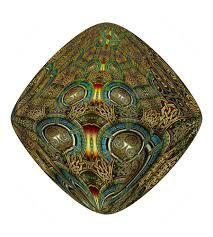# Calculate 2556

Calculate the arm size b of the trapezoid ABCD if a = 12 cm, c = 4 cm, d (AC) = d (BC) and the area S (triangle ABC) = 9 cm square.

b =  6.1847 cm

### Step-by-step explanation:

Try calculation via our triangle calculator.Did you find an error or inaccuracy? Feel free to write us. Thank you!

Tips for related online calculators